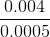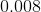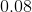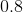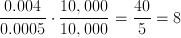## Example Questions

### Example Question #1 : How To Divide Decimals

simplify: 0.2 ÷ 0.04

5
0.005
0.05
2
0.02
Explanation:

0.2 ÷ 0.04  <- easiest way to approach is to move the decimal to the right (the same amount of spaces on each side) so that the decimal disappears

since 0.04 has 2 spaces and 0.2 only has 1, move the decimal 2 spaces to the right on each side

0.2 ÷ 0.04 = 20 ÷ 4 = 5

### Example Question #1 : How To Divide Decimals

If the cost of 20 equally priced pencils cost $5.80, then at the same rate what is the greatest number of pencils you can purchase for$50?

200

172

150

173

172.41

172

Explanation:

Remeber for this problem that you cannot spend more than $50. First find the price of each pencil by 5.80/20 = 29 cents. And then use this to do 50/0.29 = 172.41. So the greatest number of pencils you can purchase for$50 is 172 pencils.

### Example Question #3 : How To Divide Decimals

Charlie has charges on his credit card of $63.70,$37.32, and $81.78. If he pays down half his bill at once, and then pays the rest in four equal monthly installments, how much is each monthly installment? Possible Answers: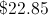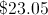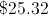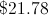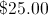Correct answer:Explanation: Adding all the charges together and dividing by 2 yields$91.40. Dividing this by 4 yields a monthly installment of \$22.85.

### Example Question #4 : How To Divide Decimals

Simplify: